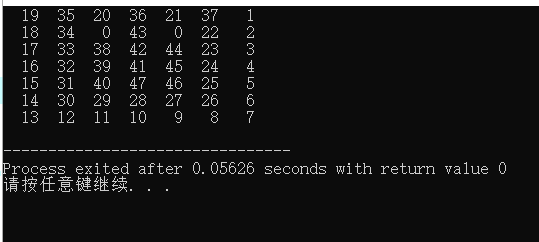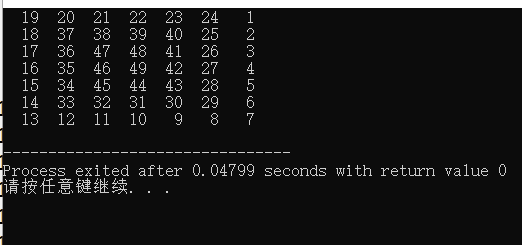++i和i++和i=i+1在比较中的区别，代码题``````#include <iostream>
#include <string.h>
int main(int argc, char** argv) {
int n;n=7;int a[n][n];memset(a,0,sizeof(a));
int step=1;
int x=0;int y=n-1;
a[y]=1;
while(step<n*n){
while(x+1<n  && !a[x+1][y]) a[++x][y]=++step;
while(y-1>=0 && !a[x][y-1]) a[x][--y]=++step;
while(x-1>=0 && !a[x-1][y]) a[--x][y]=++step;
while(y+1<n  && !a[x][y+1]) a[x][++y]=++step;
}
for(x=0;x<n;x++){
for(y=0;y<n;y++){
printf("%4d",a[x][y]);
}
printf("\n");
}
return 0;
}
``````

1个回答

while(y+1<n && !a[x][y+1])，该语句中y的值没有改变；

while(x-1>=0 && !a[x-1][y]) a[--x][y]=++step;
while(y+1 可改为
while(++x while(--y>=0 && !a[x][y]) a[x][y]=++step;
while(--x>=0 && !a[x][y]) a[x][y]=++step;
while(++y<n && !a[x][y]) a[x][y]=++step;